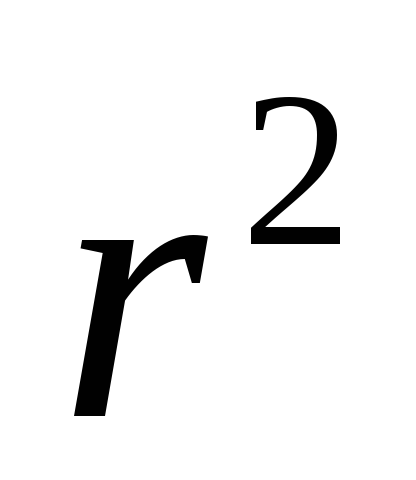# Algebra II project depreciation of Automobiles

 Date 20.05.2018 Size 40.92 Kb. #50008

Gretchen Shaffer

Morgantown High School

Monongalia County Schools

ALGEBRA II PROJECT

Depreciation of Automobiles

Unit VI: Exponential and Logarithmic Functions
You have been studying different types of functions and their real-life applications. For this project, you will research the depreciation of an automobile. You will then use that information to fit a mathematical model to data and predict the value of an automobile in 2017.
DIRECTIONS

1. Define the word “depreciation” using a dictionary.

2.) Visit www.safecarguide.com/gui/aff/expenses.htm to read about the additional expenses and depreciation of automobiles. Based on what you read, does car depreciation appear to be linear? Write a paragraph justifying why you believe that the depreciation of an automobile is or is not linear.

1. Find the price of a new Chevrolet Cavalier, Ford Mustang, and Dodge Ram. You may use the Internet, a newspaper advertisement, or correspondence with a car dealer. Then, go to http://www.dod.mil/mapsite/cardep.html. This site is a depreciation calculator. It provides information as to the value of an automobile x number of years old. Find the value of a Chevrolet Cavalier, Ford Mustang, and Dodge Ram after depreciation. Scroll to the bottom of the page. For all three types of automobiles, you will assume that you purchased them new, so enter “0” for “Years Old When Purchased.” Copy the table below and then use the information provided in addition to the depreciation calculator to find the value of each automobile at the year indicated.

 Year of Model Age of Automobile Estimated Value of the Cavalier Estimated Value of the Mustang Estimated Value of the Ram 2008 0 2009 1 2010 2 2011 3 2012 4 2013 5 2014 6 2015 7 2016 8 2017 9

1. On graph paper, plot all three sets of data on the same scatter plot. Label the axes and use the following key: red represents the Cavalier’s data, blue represents the Mustang’s data, and green represents the Ram’s data.

1. Use your graphing calculator to determine a line/curve of best fit to model the depreciation of each type (you will have three separate regression equations) of automobile. Test the following regression equations: linear, quadratic, cubic, quartic, exponential, and power. Justify the equation that you select for each model using your research, your observation of the data, and the correlation coefficients (thevalue).

1. Use the line/curve of best fit for each vehicle to predict the value of the 2008 Cavalier, the 2008 Ford Mustang and the 2008 Dodge Ram in 2018. Show your work (as you substituted into each equation) for each vehicle.

1. Your grandparents in another state have told you that they are thinking about buying you a car for your 18th birthday. However, they are not certain whether to buy you a lower-priced new car or a higher-priced used car. Based on your findings in this project, write them a letter expressing your opinion towards the price of automobiles in relation to their value through the years as they depreciate. Be sure to include whether you think it is worth it to purchase a \$25,000 vehicle with an average payment of \$500 per month for 5 years? Justify your opinion using mathematics. (*NOTE: You must follow the proper etiquette for writing a letter, such as including the date, greeting, body, closing, and signature.)

ALGEBRA II PROJECT

Depreciation of Automobiles

Unit VI: Exponential and Logarithmic Functions
Definition of Depreciation ______/2

1. Dictionary Definition of Depreciation (2 POINTS)

Paragraph Discussing Appropriate Mathematical Model for

Depreciation (Based on Research) ______/5

1. Paragraph Includes Justification of the Appropriateness of a

Linear Model vs. a Different Type of Model by Referencing

the Research (4 POINTS)

b.) Grammar, Mechanics, Punctuation, and Spelling (1 POINT)
Table of Automobile Values After Depreciation ______/8

1. Table is Correctly Filled In Based on Depreciation Calculator (8 POINTS)

Scatter Plot _____/10

1. Points Plotted Correctly for Each Type of Automobile (4 POINTS)

2. Axes Labeled Correctly (3 POINTS)

3. Key Included (3 POINT)

Line/Curve of Best Fit _____/10

1. Mathematical Model is Correct for Each Automobile (7 POINTS)

2. Justification Appropriately Supports Selection of Model (3 POINTS)

Prediction of Automobile Values in 2018 ______/5

1. Prediction of Automobile Values is Correct Based on Model (3 POINTS)

2. Work is Shown for Calculations (2 POINTS)

Letter to Grandparents Expressing Opinion Concerning Price of Automobiles ______/12

1. Letter Includes Justified Opinion as to the Prices of Today’s

Automobiles (8 POINTS)

1. Grammar, Mechanics, Punctuation, and Spelling (2 POINTS)

2. Appropriate Format for a Letter is Followed (2 POINTS)

TOTAL _____/50